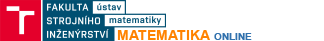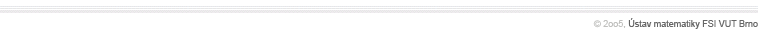Select example:

Definition area ( two dimensional interval [a,b]x[cd] )
a =  b =
c =  d =

Division count on axis ( max 50 ):
x y   z
f(x,y) =